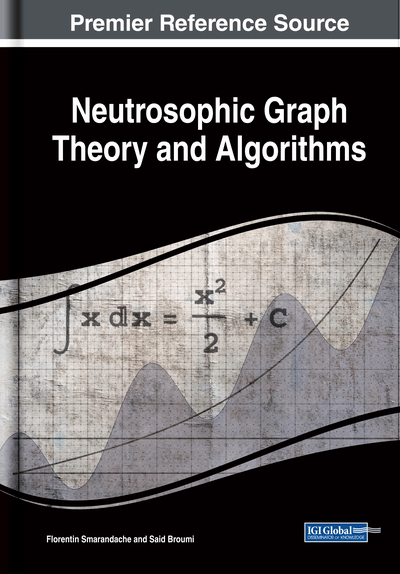# Minimal Spanning Tree in Cylindrical Single-Valued Neutrosophic Arena

Avishek Chakraborty (Narula Institute of Technology, India)
DOI: 10.4018/978-1-7998-1313-2.ch009
Available
\$37.50
No Current Special Offers

## Abstract

In this chapter, the concept of cylindrical single-valued neutrosophic number whenever two of the membership functions, which serve a crucial role for uncertainty conventional problem, are dependent to each other is developed. It also introduces a new score and accuracy function for this special cylindrical single valued neutrosophic number, which are useful for crispification. Further, a minimal spanning tree execution technique is proposed when the numbers are in cylindrical single-valued neutrosophic nature. This noble idea will help researchers to solve daily problems in the vagueness arena.
Chapter Preview
Top

## 1. Introduction

The idea of vagueness theory was first invented by (Zadeh, 1965) in his paper. After that, the paper of (Atanassov, 1986) presents the remarkable concept of an intuitionistic fuzzy set in the field of uncertainty theory in which the perception of membership function and non-membership function both are considered. Day by day, as development goes on, researchers invented triangular (Chen S., 1994)and (K.K.Yen, S.Ghoshray, & G.Roig, 1999), trapezoidal (Chen & Chen, 2007) and (S.Abbasbandy & T.Hajjari, 2009), pentagonal (R.Helen & G.Uma, 2015)and (Chakraborty A., et al., 2018) and Later (Maity, Chakraborty, Dey, Mondal, & Alam, 2018) invented heptagonal fuzzy number which are useful and plays a key role in mathematical modeling and statistical computational problem. Further, (F & XH, 2007), developed the concept of triangular intuionistic fuzzy set and (Li & Chen, 2015) introduced the idea of trapezoidal intuinistic fuzzy set. Later, (Smarandache, 1998)) invented the concept of neutrosophic set where there are three disjunctive components are considered namely i) truth, ii) indeterminacy, iii)falsity. Neutrosophic concept is a very effective & an useful idea in real life problems. Later, (Chakraborty A., Mondal, Ahmadian, Senu, Alam, & Salahshour, 2018) developed different form of triangular neutrosophic number and its application. Recently, (Chakraborty A., et al., 2019) introduced the concept of triangular bipolar neutrosophic number and its application in multi criteria decision making problem. It was defined that in case of neutrosophic set, the sum of the truth function, falsity function and hesitation function is less than or equal to 2, whenever any two of them are dependent, while the third one is independentfrom them. Now, the conception of cylindrical neutrosophic single-valued number has been imported which is fur more advanced than accustomed conception of dependence neutrosophic number.

All those neutrosophic numbers whose addition of the squares of two dependent membership functions are less than or equals to 12 and independent membership function less or equals to 1 in the 1st quadrant of three dimensional co-ordinate axes are considered as cylindrical single-valued neutrosophic number. Graphically it signifies theth part of a total unit radius cylinder. Thus, the idea of cylindrical single-valued neutrosophic number or cylindrical dependence fuzzy set in neutrosophic domain is being established.

Obviously it will contain all general neutrosophic number which will satisfy cylindrical single-valued neutrosophic number. In this article, classification and operation on cylindrical single-valued neutrosophic fuzzy number and a new important score and accuracy function is being developed which plays useful roles in vagueness theory. Later, it can be applied in various fields of science and engineering problems.

## Key Terms in this Chapter

Uncertainty: The word uncertainty means vagueness. It occurs only when the boundary of a piece of information is not clear-cut. The concept of uncertainty was first introduced by L.A. Zadeh in his research paper in 1965 as an extension of classical notion of set.

Tree: A connected graph G having no circuit is called a tree.

Membership Function: Let, A be a set, then membership function on A is a mapping from A to real interval [0,1]. The membership function of a fuzzy set is generally denoted by . For any element in , the value of generally called the membership degree of in .

Spanning Tree: A tree is T said to be a spanning tree of a connected graph G if T is a subgraph of G and T contains all the vertices of G.

Minimal Spanning Tree: A spanning tree having the smallest weight in G is called a minimal spanning tree.

Multi-Criteria Decision-Making Problem: Multi criteria decision making problem is concerned with structuring and solving decision and planning problems involving multiple criteria. The purpose is to support decision-makers facing such problems. Typically, there does not exist a unique optimal solution for such problems and it is necessary to use decision-maker's preferences to differentiate between solutions.

## Complete Chapter List

Search this Book:
Reset